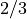Time-Edit Variables

Deprecated Since Version 15.0.0: Coding lost.

A record is automatically made every dump cycle of the values of the following non-zonal “time-edit” variables. These records are stored in the second half of the ‘.lib’ file and are accessed by the timeplot and look commands (see Chapter XXX). There are nine dummy variables holding space for future time edit variables, but even more could be added if the value of LENTDUMP in DUMPQ were increased.

Time-Edit Variable List

• ncyc KEPLER cycle number.

• jdtc Zone limiting the timestep.

• iter Number of iterations taken for convergence.

• jrn Number of zones.

• jrncalc Number of zones actually updated in the calculation (see jmcalc (p 162))

• nylib Number of distinct mass coordinates used so far in describing zoning.

• ndatq Number of post-processor-dump variables.

• dt KEPLER timestep (sec).

• radius Photospheric radius (cm) corresponding to radius withoptical depth.

• tetr Effective surface temperature (K).

• xlum Surface luminosity in electromagnetic radiation (erg/s).

• dnl Central density (g/cc).

• tnl Central temeprature (K).

• xlumn Neutrino luminosity (erg/s).

• ensc Total energry deposited so far from input “source” (ergs). (See xlum0 (p 62)).

• eni Total internal energy (ergs).

• enk Total kinetic energy (ergs).

• enp Total potential energy (ergs).

• ent Total current energy (ergs) = eni + enk + enp.

• epro Total energy produced so far by nuclear reactions less neutrino losses (ergs).

• enes Total non-neutrino energy that has so far escaped from the star’s surface (ergs).

• enc Energy check (ergs).

• enscd Rate of energy deposition by input “source” (erg/s). (See xlum0 (p 62)).

• enid Rate of change in the total internal energy (erg/s).

• enkd Rate of change in the total kinetic energy (erg/s).

• enpd Rate of change in the total potential energy (erg/s).

• entd Rate of change in the total energy (erg/s).

• eprod Total rate of nuclear energy production (erg/s).

• enesd Total rate of energy escape from the star (erg/s).

• encd Total rate of change in the energy check (erg/s).

• snuc Current nuclear energy generation rate from last call to subroutine SDOT (erg/g/s). Note that this rate applies only to the zone specified in that call.

• snl Central rate of energy production (erg/g/sec).

• snnl Central rate of nuclear energy generation (erg/g/s).

• pnl Central pressure (erg/cc).

• etal Central degeneracy parameter.

• yel Central electron abundance (moles/g).

• sigl Central entropy (k/baryon). :

• cmptime Total computer time used since generation (sec).

• totm Total amount ofma:;s in the problem (incl. summ0 (p 61)) (g).

Dummy Time-Edit Variables

• d2 Dummy variable.

• d3 Dummy variable.

• d4 Dummy variable.

• d5 Dummy variable.

• d6 Dummy variable.

• d7 Dummy variable.

• d8 Dummy variable.

• d9 Dummy variable.